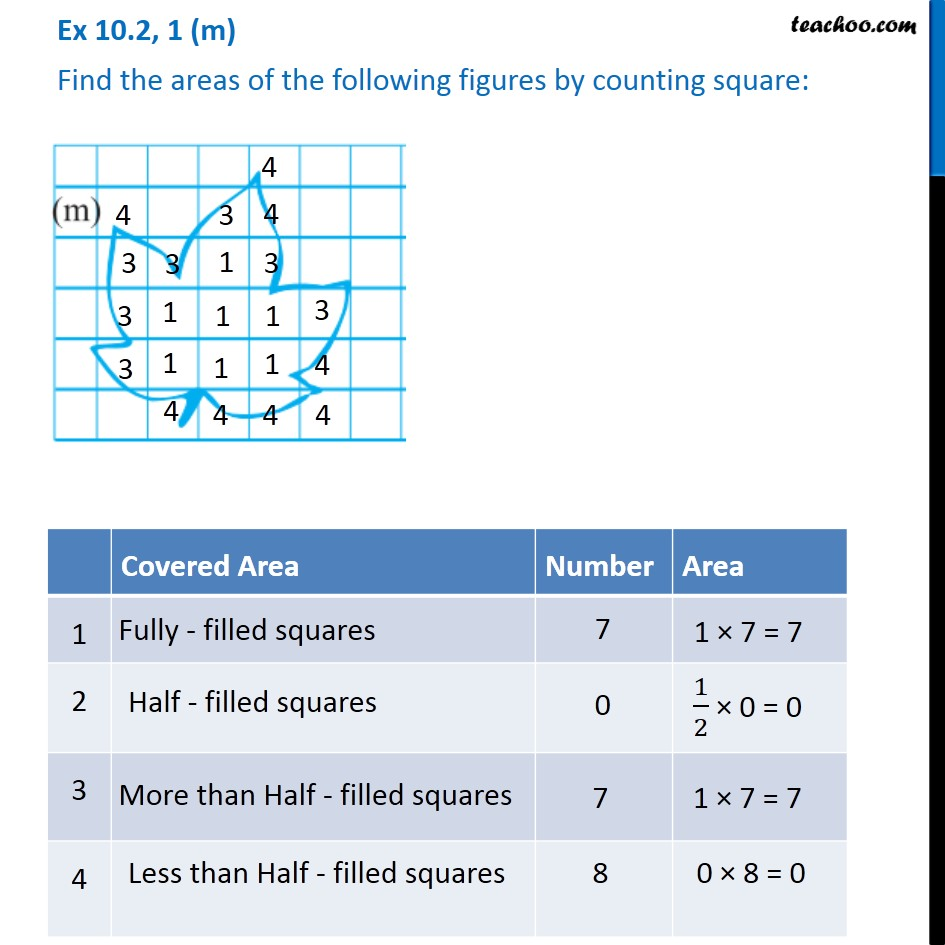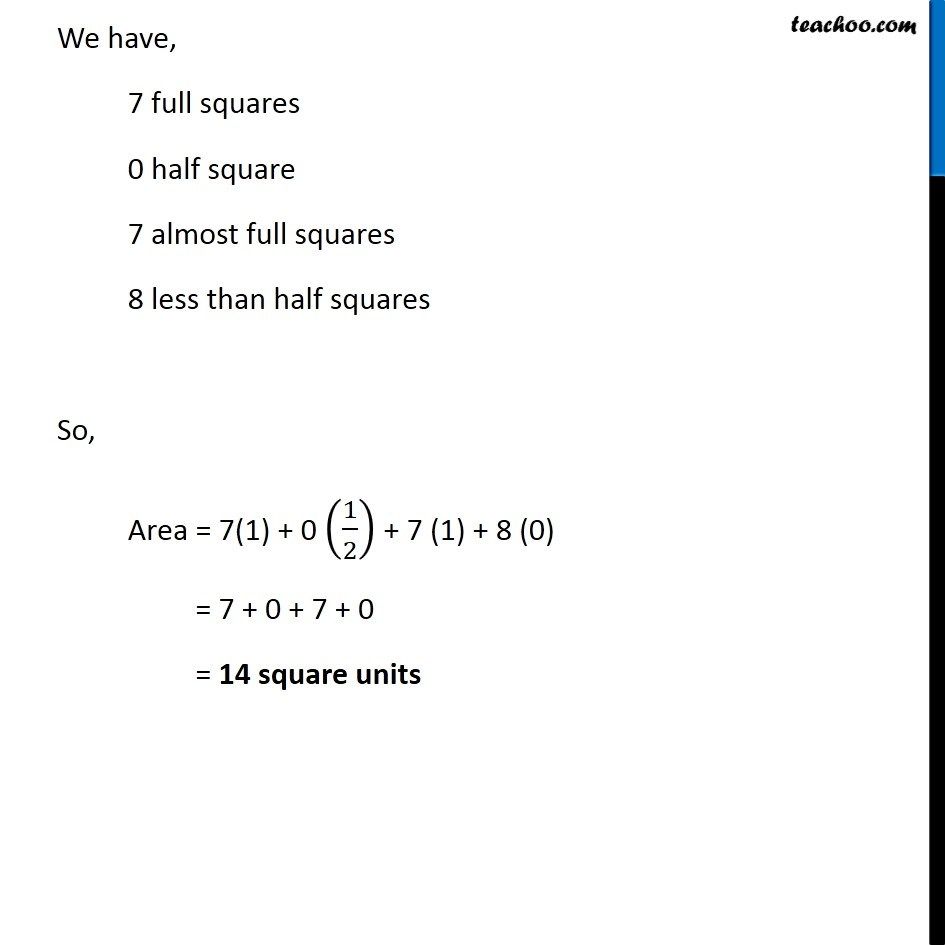Ex 10.2

Chapter 10 Class 6 Mensuration
Serial order wiseLearn in your speed, with individual attention - Teachoo Maths 1-on-1 Class

### Transcript

Ex 10.2, 1 (m) Find the areas of the following figures by counting square: Covered Area Number Area 1 Fully filled squares 7 1 × 7 = 7 2 Half filled squares 0 1/2 × 0 = 0 3 More than Half filled squares 7 1 × 7 = 7 4 Less than half filled squares 8 0 × 8 = 0 We have, 7 full squares 0 half square 7 almost full squares 8 less than half squares So, Area = 7(1) + 0 (1/2) + 7 (1) + 8 (0) = 7 + 0 + 7 + 0 = 14 square units